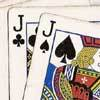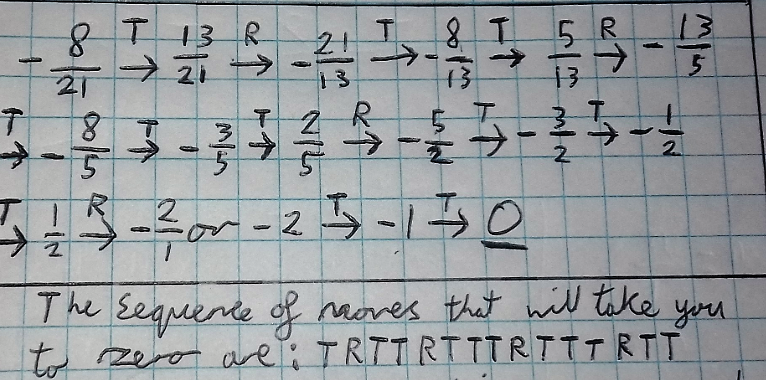#### You may also like### Do Unto Caesar

At the beginning of the night three poker players; Alan, Bernie and Craig had money in the ratios 7 : 6 : 5. At the end of the night the ratio was 6 : 5 : 4. One of them won $1 200. What were the assets of the players at the beginning of the evening?### Plutarch's Boxes According to Plutarch, the Greeks found all the rectangles with integer sides, whose areas are equal to their perimeters. Can you find them? What rectangular boxes, with integer sides, have their surface areas equal to their volumes?### 3388 Using some or all of the operations of addition, subtraction, multiplication and division and using the digits 3, 3, 8 and 8 each once and only once make an expression equal to 24. # Twisting and Turning ##### Age 11 to 14 Challenge Level: Ben from the UK, Arkadiusz from the Costello School in the UK and Ved from WBGS in the UK worked out how the rope ends up after the series of moves Twist, twist, twist, turn, twist, twist, twist, turn, twist, twist, twist, turn Ben wrote: Having explored the use of twisting and turning I used the example given to help me solve the first problem. I found an answer of$-\frac8{21}$having gone through the sequence of$1,2,3,-\frac13,\frac23,1 \frac23,2 \frac23,-\frac38,\frac58,1 \frac58,2
\frac58$to finally get to$-\frac8{21}$Arkadiusz and Ved also worked out how to get back to$0$. This is Arkadiusz' work:Mohit from Burnt Mill Academy, Harlow in the UK untangled ropes starting from a different state: TANGLE :$-\frac{11}{30}$UNTANGLE:$-\frac{11}{30}+1=\frac{19}{30}, \\
\frac{19}{30} \rightarrow -\frac1x=-\frac{30}{19},\\
-\frac{30}{19}+1=-\frac{11}{19},\\
-\frac{11}{19}+1=\frac8{19},\\
\frac8{19}\rightarrow -\frac1x=-\frac{19}8, \\
-\frac{19}8+1=-\frac{11}8, \\
-\frac{11}8+1=-\frac38, \\
-\frac38+1=\frac58,\\
\frac58\rightarrow-\frac1x= -\frac85,\\
-\frac85+1=-\frac35, \\
-\frac35+1=\frac25,
\frac25\rightarrow-\frac1x=-\frac52, \\
-\frac52+1=-\frac32,\\
-\frac32+1=-\frac12,\\
-\frac12+1=\frac12, \\
\frac12\rightarrow-\frac1x=-2, \\
-2+1=-1, \\
-1=1=0\$
T,R,T,T,R,T,T,T,R,T,T,R,T,T,T,R (T,T)

Arkadiusz described a method to untangle ropes starting from any position:

We keep twisting until we get a positive value, then we turn and repeat over and over until we get zero.